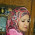## 23.11.14

### OOP : Excercise-9 : Program to find area of triangle, circle, and rectangle using function overloading

Write a C++ program to find area of triangle, circle, and rectangle using function overloading

1.#include
using namespace std;
class printdata
{
public:
float pi=3.14;
void print(float a,float b,float h)
{
cout<< "Area of tringle: "<< a*b/h;
}
void print(float b,float l)
{
cout<<"Area of rectangle: "<<b*l;
}
void print(float r)
{
cout<< "Area of circle: "<<pi*r*r;
}
};
int main()
{
printdata pd;
pd.print(5.0,5.5,2);
pd.print(3.5,4.5);
pd.print(3.3);
}

1.noted and found correct

2.plz other program

2.#include
using namespace std;
class print
{
public:
const float pi=3.1416;
void area(float b,float h,float d)
{
cout<<"Area of triangle:\n"<<(b*h)/d;
}
void area(float r)
{
cout<<"\nArea of circle:\n"<<(pi*r*r);
}
void area(float l,float b)
{
cout<<"\nArea of rectangle:\n"<<(l*b);
}
};
int main()
{
print fd;
fd.area(5.5,6.5,2);
fd.area(3.0,3.5);
fd.area(2.2);
}

1.noted and found correct

3.#include
using namespace std;
class printdata
{
public:
float pi=3.14;
void print(float a,float b,float h)
{
cout<< "Area of tringle: "<< a*b/h;
}
void print(float b,float l)
{
cout<<"Area of rectangle: "<<b*l;
}
void print(float r)
{
cout<< "Area of circle: "<<pi*r*r;
}
};
int main()
{
printdata pd;
pd.print(5.0,5.5,2);
pd.print(3.5,4.5);
pd.print(3.3);
}

1.4.#include
using namespace std;
class pr
{
public:
float p=3.1416;
void print(float b,float h,float div)
{
cout<<"the area of triangle is :"<>x;
cin>>y;
pb.print(x,y);
float z,e,d=2;
cin>>z;
cin>>e;
pb.print(z,e,d);
float h;
cin>>h;
pb.print(h);
}

1.incomplete program. submit again.

5.dont know what the hell is going on,my full program code doesn't show up here...i tried twice but got the same problem

1.sometimes its happen due to slow internet connection. I checked it. submit again after sometimes. try in night for better speed.

6.http://itlearn24.blogspot.com/2014/11/c-program-to-find-area-of-triangle.html

1.noted and found correct

7.Name- Habibur Rahman
ID-201421091
Batch-46

#include
using namespace std;
class printdata
{
public:
float pi=3.14;
void print(float X,float Y,float i)
{
cout<< "tringle: "<< X*Y/i;
}
void print(float Y,float l)
{
cout<<"rectangle: "<<Y*l;
}
void print(float n)
{
cout<< "circle: "<<pi*n*n;
}
};
int main()
{
printdata pd;
pd.print(5.0,5.5,2);
pd.print(3.5,4.5);
pd.print(3.3);
}

1.noted. but some parts of your program are exactly matched from others program. as like:
int main()
{
printdata pd;
pd.print(5.0,5.5,2);
pd.print(3.5,4.5);
pd.print(3.3);
}

8.#include
using namespace std;

const float pi=3.14;
float area(float n,float b,float h)
{
float ar;
ar=n*b*h;
cout<<"The area of Triangle : "<>b>>h;
area(0.5,b,h);

cout<<"Enter the radius of circle :";
cin>>r;
area(r);

cout<<"Enter the Lenght & width of Rectangle: ";
cin>>l>>w;
area(l,w);
return 0;
}

9.md. kashem
45th

#include
using namespace std;
class printdata
{
public:
float pi=3.14;
void print(float s,float r,float h)
{
cout<< "the tringle: "<< s*r/h;
}
void print(float r,float l)
{
cout<<"the rectangle: "<<r*l;
}
void print(float x)
{
cout<< "the circle: "<<pi*x*x;
}
};
int main()
{
printdata pd;
pd.print(5.0,5.5,2);
pd.print(3.5,4.5);
pd.print(3.3);
}

10.https://www.dropbox.com/s/e1wl2r2qd4m3yfj/exercise09.cpp?dl=0

ID : 201510545
Name : Aubhijit Sarkar
Batch : 48th

Comment Here